edHelper.com Math Worksheets Multiplication Worksheets 3rd Grade MultiplicationWorksheets, Lessons, and Printables

Math Facts

Quick Math Facts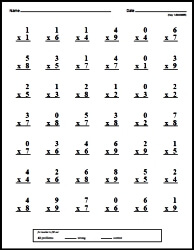Make Quick Math Facts Printable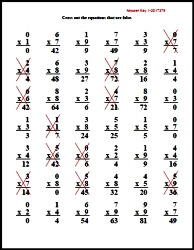Multiplication Table and Chart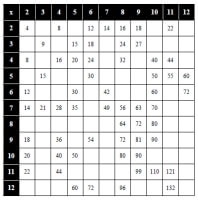Multiplication Table and Chart

Multiplication Word Problems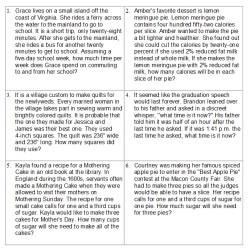Multiplication Word Problems (3rd grade) Multiplication Word Problems (4th grade) Multiplication Word Problems (5th grade) Multiplication Word Problems (6th grade)

Introduction to Multiplication (Part 1)
Multiplication Concepts (with graphics of pictures)
Write the sum and product
Write the addition and multiplication fact
Write the sum and product (smaller graphics)
Write the addition and multiplication fact (smaller graphics)
Multiplication with Rows (dot graphics)
Multiply with 2
Multiply with 3
Multiply with 4
Multiply with 5
Multiply with 6
Mixed
Write the sum and then write the product
Multiply with 2
Multiply with 3
Multiply with 4
Multiply with 5
Multiply with 6
Multiply with 7
Multiply with 8
Multiply with 9
Multiply with 10
Mixed
Addition and Multiplication: Fill in the missing numbers
Multiply with 2
Multiply with 3
Multiply with 4
Multiply with 5
Multiply with 6
Multiply with 7
Multiply with 8
Multiply with 9
Multiply with 10
Mixed
Addition and Multiplication: Comparison
Comparison
Introduction to Multiplication: Mixed Review
Introduction to Multiplication Mixed Review

Introduction to Multiplication (Part 2)
Basic Multiplication Worksheets
Multiply with 0 and 1
Multiply with 2
Multiply with 3
Multiply with 4
Multiply with 5
Multiply with 6
Multiply with 7
Multiply with 8
Multiply with 9
Multiply with 10
Mixed Basic Multiplication Worksheets (numbers 0 to 10)
Vertical multiplication
Horizontal multiplication
Vertical and horizontal multiplication
Fill in the missing number
Mixed Basic Multiplication Worksheets (numbers 2 to 10)
Vertical multiplication
Horizontal multiplication
Vertical and horizontal multiplication
Fill in the missing number
Mixed Basic Multiplication Worksheets with a smaller font (numbers 2 to 10)
Vertical multiplication
Horizontal multiplication
Multiplication Problem Solving
Multiplication problem solving
Multiplication Mixed Review
Multiplication Mixed Review

Easy Multiplication Activity Books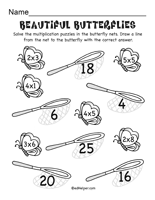Multiplication Activity Pages (book #1)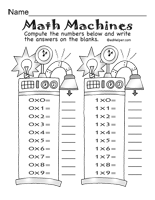Multiplication Activity Pages (book #2)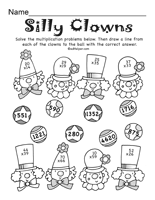Multiplication Activity Pages (book #3)

Multiplication and Division
Fill in the quotient
Fill in the missing number
Fill in the missing number

Multiplication
Column Multiplication with Extra Space To Solve Problems
1-2 digits by 1 digit column multiplication
2 digits (and increasing 1 digit every 2 rows) by 1 digit column multiplication
2 digits (and increasing 1 digit every row) by 1 digit column multiplication
Multiples of 10 multiplication (2-5 digits by 1 digit)
2 digits by 2 digits column multiplication
2-3 digits by 2 digits column multiplication
4-5 digits by 2 digits column multiplication
2 digits (and increasing 1 digit every 2 rows) by 2 digit column multiplication
2 digits (and increasing 1 digit every row) by 2 digit column multiplication

Column Multiplication (more problems with less space)
1-2 digits by 1 digit column multiplication
2 digits (and increasing 1 digit every 2 rows) by 1 digit column multiplication
2 digits (and increasing 1 digit every row) by 1 digit column multiplication
Multiples of 10 multiplication (2-5 digits by 1 digit)
2 digits by 2 digits column multiplication
2-3 digits by 2 digits column multiplication
4-5 digits by 2 digits column multiplication
2 digits (and increasing 1 digit every 2 rows) by 2 digit column multiplication
2 digits (and increasing 1 digit every row) by 2 digit column multiplication

Part 2
1-2 digits by 1 digit across multiplication
3-4 digits by 1 digit across multiplication
1-2 digits by 2 digits across multiplication
Across multiplication with 3-5 numbers
Fill in the missing numbers: 1-2 digits
Fill in the missing numbers: 2 digits
Fill in the missing numbers: 2-3 digits
Math multiplication boxes: 3 x 3 (numbers 1-9)
Math multiplication boxes: 4 x 4 (numbers 1-9)
Math multiplication boxes: 3 x 3 (numbers 4-19)
Multiplication word problems (basic)
Multiplication word problems
Multiplication word problems
Multiplication Mixed Review

Division Facts Through 10
Multiplication and Division: Fill in the missing numbers
Multiplication and division with 2
Multiplication and division with 3
Multiplication and division with 4
Multiplication and division with 5
Multiplication and division with 6
Multiplication and division with 7
Multiplication and division with 8
Multiplication and division with 9
Multiplication and division with 10
Multiplication and division (mix)

Multiplication

Have a suggestion or would like to leave feedback?